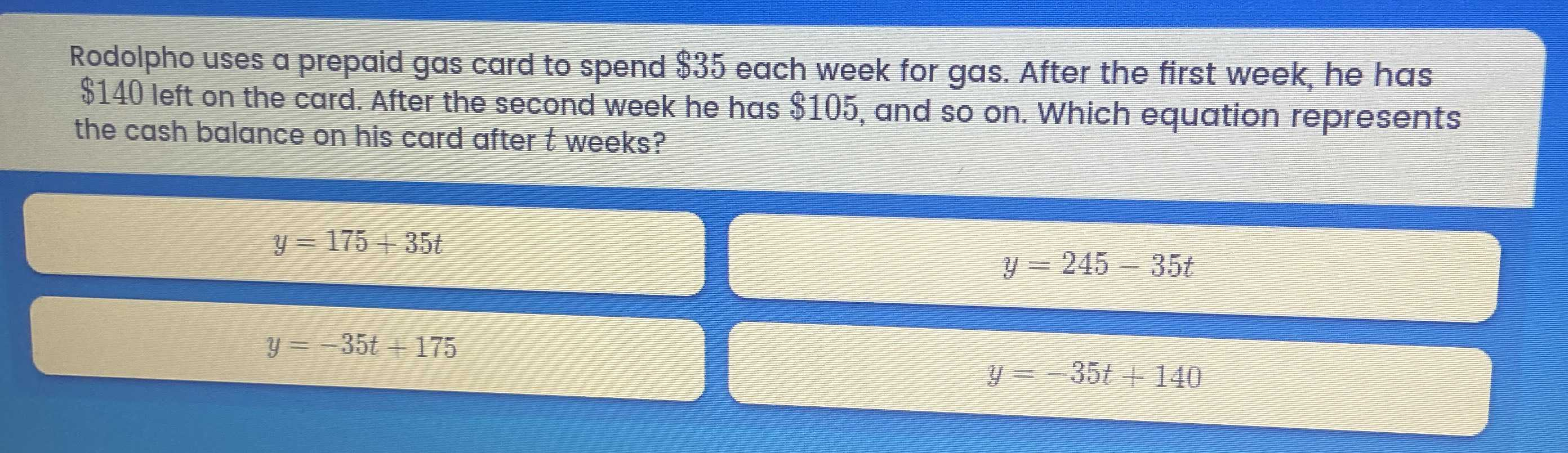### ¿Todavía tienes preguntas de matemáticas?

Pregunte a nuestros tutores expertos
Algebra
PreguntaRodolpho uses a prepaid gas card to spend $$\ 35$$ each week for gas. After the first week, he has

$$\ 140$$ left on the card. After the second week he has $$\ 105 ,$$ and so on. Which equation represents the cash balance on his card after $$t$$ weeks?

$$y = 175 + 35 t$$

$$y = - 35 t + 175$$

$$y = 245 - 35 t$$

$$y = - 35 t + 140$$